# Relation Between Continuity & Differentiability JEE Notes | EduRev

## JEE : Relation Between Continuity & Differentiability JEE Notes | EduRev

The document Relation Between Continuity & Differentiability JEE Notes | EduRev is a part of the JEE Course Mathematics (Maths) Class 12.
All you need of JEE at this link: JEE

E. Relation between Continuity & Differentiability

If a function f is derivable at x then f is continuous at x.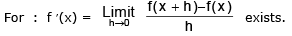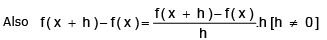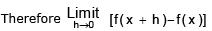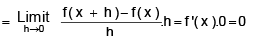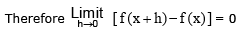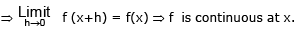If f(x) is derivable for every point of its domain, then it is continuous in that domain .

The converse of the above result is not true :

"If f is continuous at x, then f may or maynot be derivable at x"

The functions f(x) =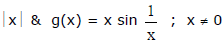& g(0) = 0 are continuous at x = 0 but not derivable at x = 0.

Remark :

(a) Let f'+(a) = p  &  f'_(a) = q  where p & q are finite then :

(i) p = q  ⇒  f  is derivable at x = a ⇒ f is continuous at x = a.

(ii) p ≠ q  ⇒  f  is not derivable at  x = a  but f is continuous at x = a

Differentiable ⇒ Continuous ; Non-differentiable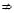Discontinuous

But Discontinuous ⇒ Non-differentiable .

(b) If a function f is not differentiable but is continuous at x = a it geometrically implies a sharp corner at x = a.

Ex.15 If f(x) =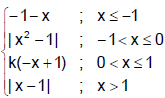, then find the value of k so that f(x) becomes continuous at x = 0. Hence, find all the points where the functions is non-differentiable.

Sol. From the graph of f(x) it is clear that for the function to be continuous only possible value of k is 1.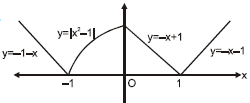Points of non-differentiability are x = 0, ±1.

Ex.16 If f(x) =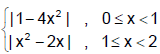where [.] denotes the greatest integer function.

Discuss the continuity and differentiability of f(x) in [0, 2).

Sol. Since 1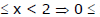x - 1 < 1 then [x2 - 2x] = [(x - 1)2 - 1] = [(x - 1)2] - 1 = 0 - 1 = -1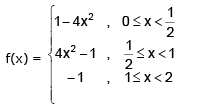Graph of f(x) :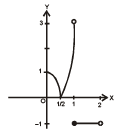It is clear from the graph that f(x) is discontinuous at x = 1 and not differentiable at  x =1/2,and x= 1

Further details are as follows :⇒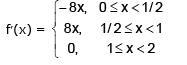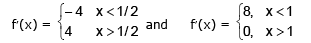Hence, which shows f(x) is not differentiable at x = 1/2 (as RHD = 4 and LHD = –4) and x = 1 (as RHD = 0 and LHD = 8). Therefore, f(x) is differentiable, for x ∈ [0, 2) - {1/2, 1}

Ex.17 Suppose f (x) =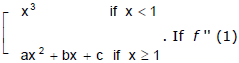. If f '' (1) exist then find the value of a2 + b2 + c2.

Sol. For continuity at x = 1 we leave f (1) = 1 and f (1+)  = a + b + c

a + b + c = 1 ....(1)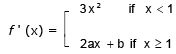for continuity of  f ' (x) at x = 1 f ' (1) = 3; f ' (1+) = 2a + b

hence 2a + b = 3 ....(2)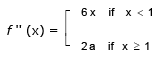f '' (1–) = 6; f '' (1+) = 2a for continuity of f '' (x) 2a = 6 ⇒  a = 3

from  (2), b = – 3 ; c = 1. Hence  a = 3, b = – 3 ; c = 1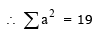Ex.18 Check the differentiability of the function f(x) = max {sin-1 |sin x|, cos-1 |sin x|}.

Sol. sin-1 |sin x| is periodic with period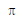⇒ sin-1 |sin x| =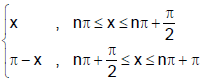Also cos-1 |sin x| =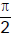- sin-1 |sin x|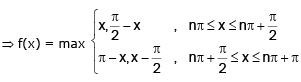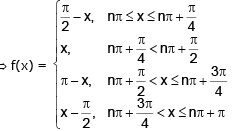⇒ f(x) is not differentiable at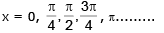⇒ f(x) is not differentiable at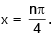Ex.19 Find the interval of values of k for which the function f(x) = |x2 + (k - 1) |x| - k| is non differentiable at five points.

Sol.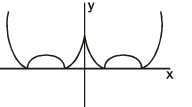f(x) = |x2 + (k – 1) |x| – k|    = |(|x| – 1) (|x| + k)|

Also f(x) is an even function and f(x) is not differentiable at five points.
So |(x – 1) (x + k)| is non differentiable for two positive values of x.

⇒ Both the roots of (x – 1) (x + k) = 0 are positive.

⇒ k < 0 ⇒ k ∈ (–∝, 0).

Definition : A function f is differentiable at a if f'(a) exists. It is differentiable on an open interval (a,b) [or (a, ∝) or (–∝, a) or (– ∝, ∝)] if it is differentiable at every number in the interval.

Derivability Over An Interval : f(x) is  said  to  be  derivable  over  an  interval  if  it  is derivable  at each & every  point of  the  interval. f(x) is said  to be derivable over the  closed interval  [a, b] if :

(i) for the points a and b, f'(a+)  &  f'(b -) exist  &

(ii) for any point c such that  a < c < b, f'(c+)  &  f'(c -) exist & are equal .

Offer running on EduRev: Apply code STAYHOME200 to get INR 200 off on our premium plan EduRev Infinity!

## Mathematics (Maths) Class 12

209 videos|222 docs|124 tests

,

,

,

,

,

,

,

,

,

,

,

,

,

,

,

,

,

,

,

,

,

;